# The Electric Field The electric field is present

• Slides: 12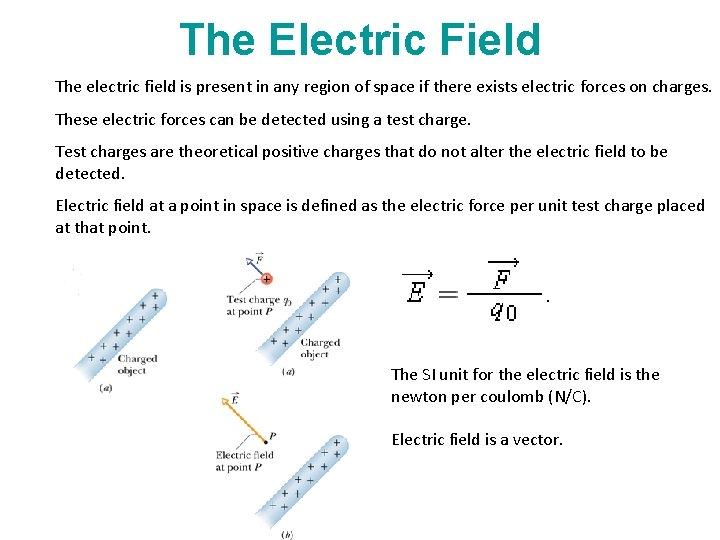The Electric Field The electric field is present in any region of space if there exists electric forces on charges. These electric forces can be detected using a test charge. Test charges are theoretical positive charges that do not alter the electric field to be detected. Electric field at a point in space is defined as the electric force per unit test charge placed at that point. The SI unit for the electric field is the newton per coulomb (N/C). Electric field is a vector.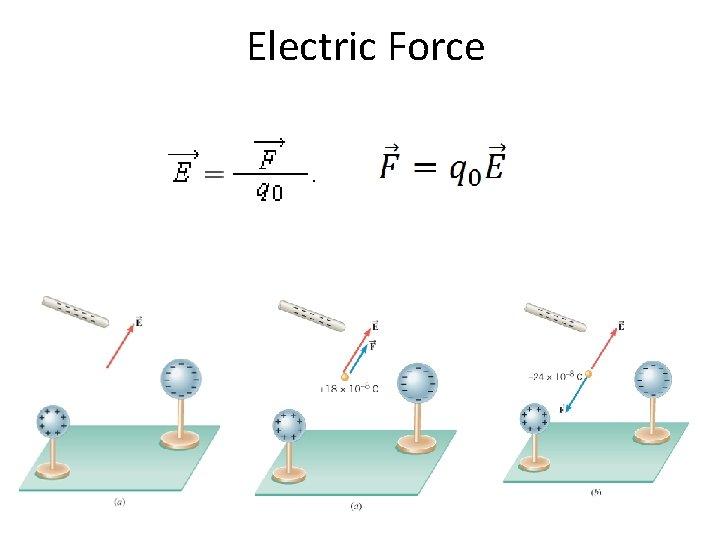Electric Force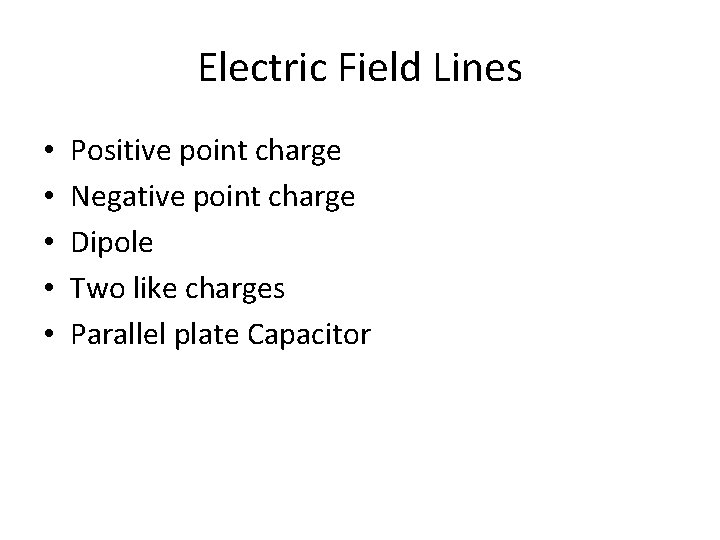Electric Field Lines • • • Positive point charge Negative point charge Dipole Two like charges Parallel plate Capacitor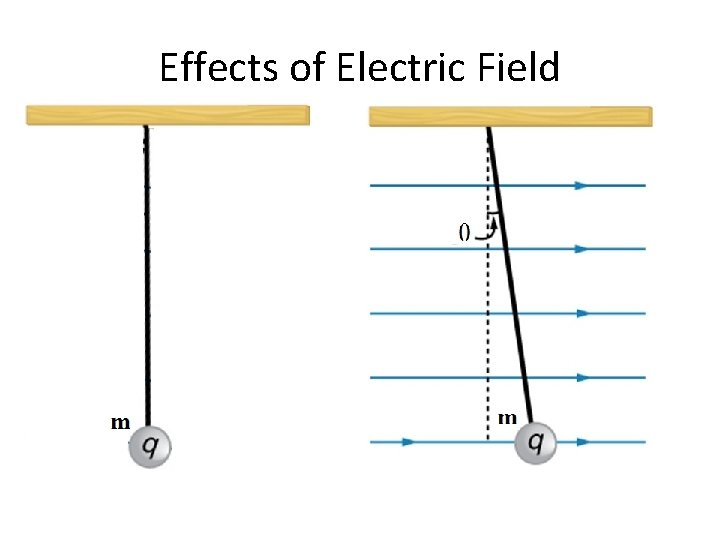Effects of Electric FieldCharge moving in an Electric Field [CJ 10 P 52, Chap 18] The right drawing shows an electron entering the lower left side of a parallel plate capacitor and exiting at the upper right side. The initial speed of the electron is 5. 71 × 106 m/s. The capacitor is 2. 00 cm long, and its plates are separated y 0. 150 cm. a. When the capacitor plates are not charged, as in the left drawing, draw the subsequent motion of the electron, and determine how long the electron takes to cross the plates. b. Assume that the electric field between the plates is uniform everywhere in the right drawing, and find its magnitude.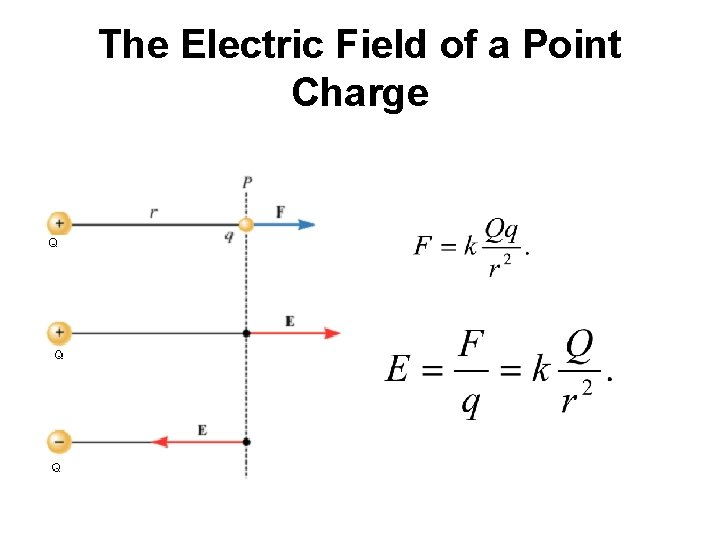The Electric Field of a Point Charge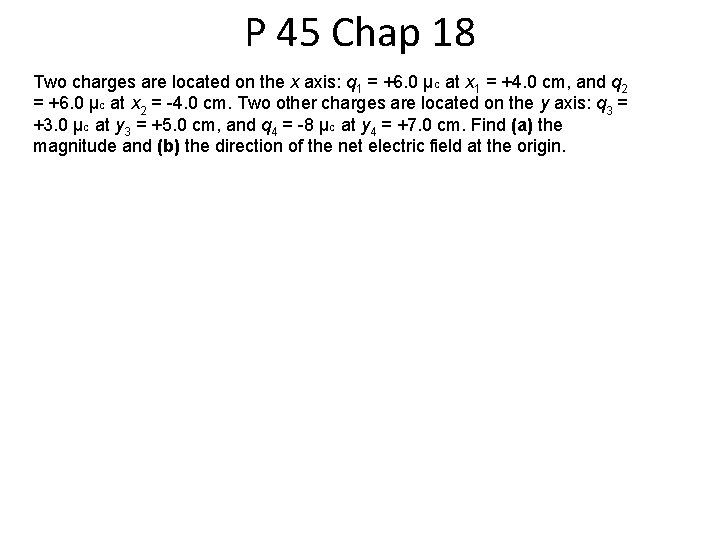P 45 Chap 18 Two charges are located on the x axis: q 1 = +6. 0 µC at x 1 = +4. 0 cm, and q 2 = +6. 0 µC at x 2 = -4. 0 cm. Two other charges are located on the y axis: q 3 = +3. 0 µC at y 3 = +5. 0 cm, and q 4 = -8 µC at y 4 = +7. 0 cm. Find (a) the magnitude and (b) the direction of the net electric field at the origin.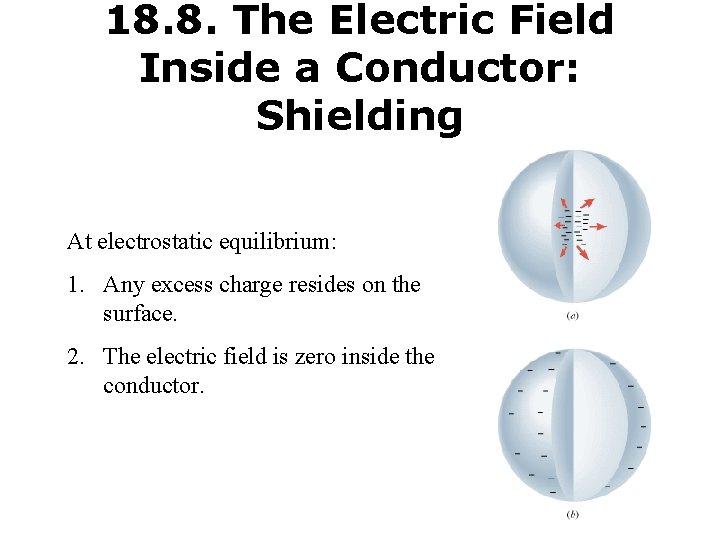18. 8. The Electric Field Inside a Conductor: Shielding At electrostatic equilibrium: 1. Any excess charge resides on the surface. 2. The electric field is zero inside the conductor.Conductor in Electric Field Under electrostatic equilibrium: 1. The conductor shields the electric field. 2. The electric field just outside the surface a conductor is perpendicular to the surface. Sensitive electronic circuits are often enclosed within metal boxes that provide shielding from external fields.18. 10 Copying MachineElectrostatic Precipitator (a) Schematic of an electrostatic precipitator. Air is passed through grids of opposite charge. The first grid charges airborne particles, while the second attracts and collects them. (b) The dramatic effect of electrostatic precipitators is seen by the absence of smoke from this power plant. (credit: Cmdalgleish, Wikimedia Commons)Gauss' Law: The electric flux, ΦE through a Gaussian surface is equal to the net charge Q enclosed by the surface divided by, ϵ 0 the permittivity of free space: